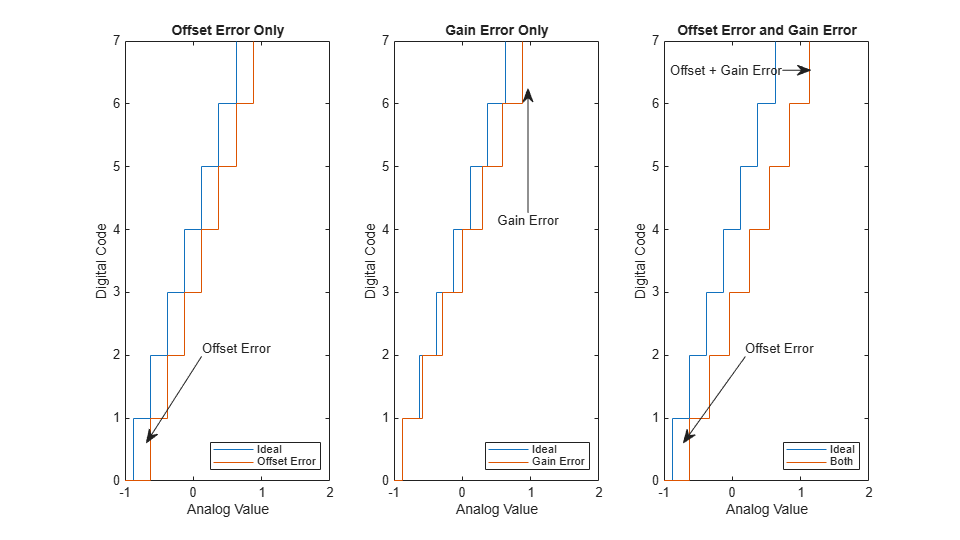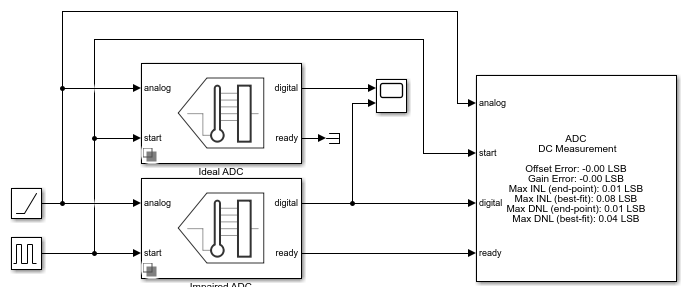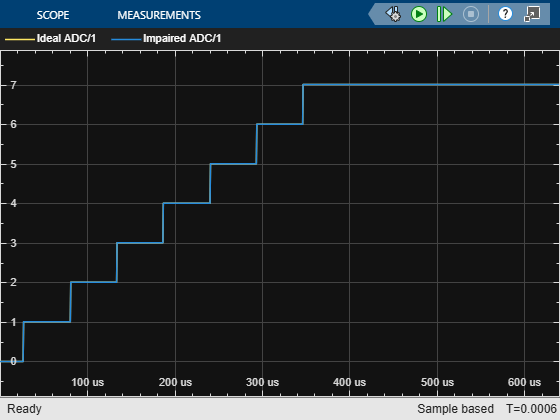## Measuring Offset and Gain Errors in ADC

This example shows how offset error and gain error are calculated and how each affects the transfer curve of an ADC. Offset error and gain error characterize part of the linearity error in an ADC.

Offset error is the difference between the center of the least significant code and the center of the same code on an ideal ADC with the same number of bits. Offset error is usually reported in units of the least significant bit (LSB) of the converter. One LSB is equivalent to the converter's quantization interval.

Gain error in LSB is the difference between the center of the most significant code after offset error correction and the center of the same code on an ideal ADC with the same number of bits.

Due to the difficulty in determining the precise location of the center of a code with a non finite boundary, these values are most commonly measured with respect to the first and last threshold of the converter, respectively.

### Units for Offset Error and Gain Error

The unit, which is used in this example, is defined as:

``

Thus, an error in(volts) translates intoas follows:

``

whereis the full scale range of the ADC andis the number of bits of the ADC.

Linearity errors are also commonly reported in normalized units with respect to the full scale range:

``

Another unit sometimes used for linearity errors used is percent full scale. Percent full scale is defined as normalized units multiplied by 100:

``

### Calculate Offset Error and Gain Error

Use a 3-bit ADC with dynamic range [-1 1]. Define an offset error of `1` LSB and a gain error of `1` LSB.

```Nbits = 3; Range = [-1 1]; % ADC Full Scale Range OffsetError = 1; GainError = 1; LSB = (max(Range) - min(Range)) / (2^Nbits - 1); ```

The digital codes from the ideal ADC are identical to those from the experimental ADCs.

```[TC0Analog, TC0Digital] = og2tc(Nbits, Range, 0, 0); % Ideal ADC Transfer Curve TC1Analog = og2tc(Nbits, Range, OffsetError, 0); % Offset Error Only TC2Analog = og2tc(Nbits, Range, 0, GainError); % Gain Error Only TC3Analog = og2tc(Nbits, Range, OffsetError, GainError); % Both Offset Error and Gain Error plotAdcTcForExample(TC0Digital, TC0Analog, TC1Analog, TC2Analog, TC3Analog, Nbits); ```The sum of offset error and gain error is known as full-scale error. In monopolar converters zero error, essentially offset error defined at analog level `0`, is identical to regular offset error. In bipolar converters such as those above, offset error and zero error are different quantities.

### Use of Linearity Errors as Impairments

Compare a flash ADC with offset and gain error to one with no impairments.

```model = 'OffsetGainExample'; open_system(model); open_system([model '/Scope']); sim(model); ```The ADC DC Measurement block confirms the values of the impairments to within a margin of error determined by the sample rate of the system. The offset error and gain error were both entered as `1` LSB. Offset error was measured as 1.08 LSB and gain error was measured as 0.97 LSB. Errors in these measurements are due to the converter not sampling exactly at its threshold values. The maximum expected error in LSB is:

``

whereis the slope of the input ramp/sawtooth signal in LSB/s, andis the ADC's conversion start frequency.

##### SupportGet trial now